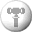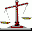Size : 327 KBfunwithfigures.comQB - Math Practice Multiplication, Addition, Subtraction and Division.Math Rescue Mathematical game to improve your skills in addition, subtraction, multiplication and division.Multiplication Facts Practice times tables with digits 0 to 12.BunnyMath (For PocketPC) A fun way for children to learn math. The pocketPC version actually speaks.Balance Keeper Helps children practice addition, subtraction, logical thinking, basic algebra.